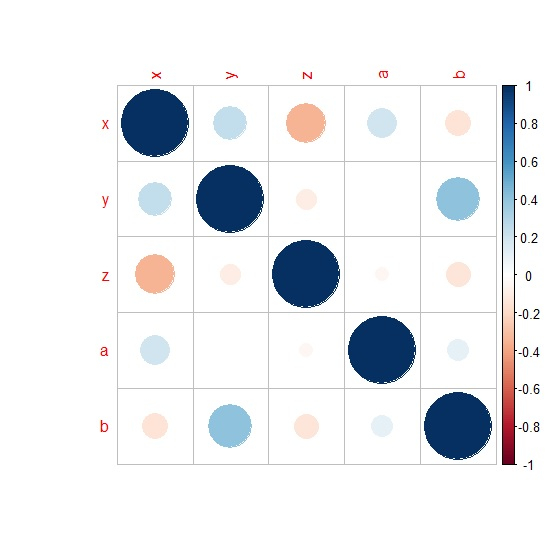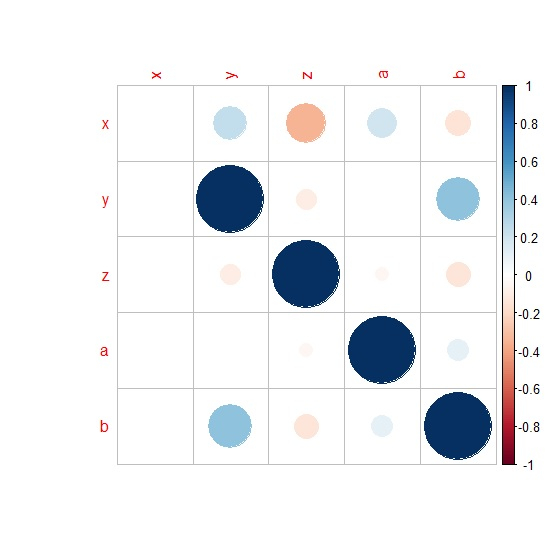# How to disable the display of some correlations using corrplot in R?

R ProgrammingServer Side ProgrammingProgramming

When we create a correlation plot using corrplot the correlation among variables is displayed on the plot, if we want to disable some of those correlations then we first need to set them to NA in the correlation matrix and then use the corrplot function with na.label set to blank as " ".

Check out the below given example to understand how it works.

## Example

Following snippet creates a sample data frame −

x<-rpois(20,5)
y<-rpois(20,1)
z<-rpois(20,2)
a<-rpois(20,5)
b<-rpois(20,2)
df<-data.frame(x,y,z,a,b)
df

## Output

The following dataframe is created −

   x  y  z a  b
1  7  1  3 8  2
2  6  0  2 9  1
3  7  3  0 4  4
4  3  1  3 5  4
5  3  1  0 6  5
6  4  0  4 3  0
7  3  3  5 3  3
8  8  1  0 1  1
9  8  1  2 7  0
10 6  4  3 5  4
11 4  0  5 5  4
12 1  0  5 4  1
13 6  2  0 7  2
14 5  2  5 5  0
15 3  0  1 3  1
16 5  0  1 2  1
17 4  1  2 5  2
18 3  1  2 6  1
19 6  0  5 5  2
20 5  1  1 7  3

To create correlation matrix for data in df, add the following code to the above snippet −

x<-rpois(20,5)
y<-rpois(20,1)
z<-rpois(20,2)
a<-rpois(20,5)
b<-rpois(20,2)
df<-data.frame(x,y,z,a,b)
Corr_M<-cor(df)
Corr_M

## Output

If you execute all the above given snippets as a single program, it generates the following output −

      x            y             z            a           b
x   1.0000000   0.24491966  -0.34910183   0.19145954  -0.1414168
y   0.2449197   1.00000000  -0.09383816   0.00000000   0.4081691
z  -0.3491018  -0.09383816   1.00000000  -0.04151439  -0.1358051
a   0.1914595   0.00000000  -0.04151439   1.00000000   0.1013302
b  -0.1414168   0.40816908  -0.13580512   0.10133025   1.0000000

To load corrplot package and create corrplot of correlation matrix Corr_M, add the following code to the above snippet −

library(corrplot)
corrplot(Corr_M)

## Output

If you execute all the above given snippets as a single program, it generates the following output −To set the first column of Corr_M to NA, add the following code to the above snippet −

Corr_M[,1]<-NA
Corr_M

## Output

If you execute all the above given snippets as a single program, it generates the following output −

    x     y             z           a             b
x  NA   0.24491966  -0.34910183   0.19145954  -0.1414168
y  NA   1.00000000  -0.09383816   0.00000000   0.4081691
z  NA  -0.09383816   1.00000000  -0.04151439  -0.1358051
a  NA   0.00000000  -0.04151439   1.00000000   0.1013302
b  NA   0.40816908  -0.13580512   0.10133025   1.0000000

To create corrplot of Corr_M again to visualize the correlation plot without first column, add the following code to the above snippet −

corrplot(Corr_M,na.label=" ")


## Output

If you execute all the above given snippets as a single program, it generates the following output −